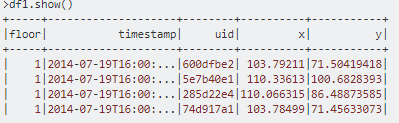# Best way to get the max value in a Spark dataframe column

1 view

I'm trying to figure out the best way to get the largest value in a Spark dataframe column.

Consider the following example:

```df = spark.createDataFrame([(1., 4.), (2., 5.), (3., 6.)], ["A", "B"])
df.show()```

Which creates:

```+---+---+
|  A|  B|
+---+---+
|1.0|4.0|
|2.0|5.0|
|3.0|6.0|
+---+---+```

My goal is to find the largest value in column A (by inspection, this is 3.0). Using PySpark, here are four approaches I can think of:

```# Method 1: Use describe()
float(df.describe("A").filter("summary = 'max'").select("A").collect().asDict()['A'])

# Method 2: Use SQL
df.registerTempTable("df_table")
spark.sql("SELECT MAX(A) as maxval FROM df_table").collect().asDict()['maxval']

# Method 3: Use groupby()
df.groupby().max('A').collect().asDict()['max(A)']

# Method 4: Convert to RDD
df.select("A").rdd.max()```

Each of the above gives the right answer, but in the absence of a Spark profiling tool I can't tell which is best.

Any ideas from either intuition or empiricism on which of the above methods is most efficient in terms of Spark runtime or resource usage, or whether there is a more direct method than the ones above?

by (13.2k points)

All the methods you have described are perfect for finding the largest value in a Spark dataframe column.

Methods 2 and 3 are almost the same in terms of  physical and logical plans. Method 4 can be slower than operating directly on a DataFrame. Method 1 is somewhat equivalent to 2 and 3.

There is another more efficient method, whose format is the same as method 3 .

df.groupby().max('A').collect().['max(A)']

Only difference from method 3 is that asDict() is missing.

by (17k points)
Very well explained. Thank you.
by (107k points)
Understood the concepts properly thanks
by (44.6k points)

A particular column's MAX value of a dataframe can be determined using this:

max_value = df.agg({"any-column": "max"}).collect()

by (29.1k points)
I would prefer this answer to be accepted.
Note:   must be in the command to get the result.
by (63.4k points)
by (95.5k points)>row1 = df1.agg({"x": "max"}).collect()

>print row1

Row(max(x)=110.33613)

>print row1["max(x)"]

110.33613

The answer is almost the same as method3. but seems the "asDict()" in method3 can be removed.

by (47.2k points)
Max value for a particular column of a dataframe can be achieved by using -

your_max_value = df.agg({"your-column": "max"}).collect()
by (31.8k points)

If you're looking to do this directly using Scala (using Spark 2.0.+), you do it like this:

scala> df.createOrReplaceTempView("TEMP_DF")
scala> val myMax = spark.sql("SELECT MAX(x) as maxval FROM TEMP_DF").
collect()(0).getInt(0)
scala> print(myMax)
117
by (29.8k points)
I tried it like this .. this worked for me thankyou
by (33.2k points)
edited by

In PySpark, you can do this simply by using this code:

max(df.select('ColumnName').rdd.flatMap(lambda x: x).collect())

by (40.4k points)

Try using the code mentioned below:

df.select(f.max(f.col("A")).alias("MAX")).limit(1).collect().MAX

For my data, I got the following benchmarks:

df.select(f.max(f.col("A")).alias("MAX")).limit(1).collect().MAX

CPU times: user 2.31 ms, sys: 3.31 ms, total: 5.62 ms

Wall time: 3.7 s

df.select("A").rdd.max()

CPU times: user 23.2 ms, sys: 13.9 ms, total: 37.1 ms

Wall time: 10.3 s

df.agg({"A": "max"}).collect()

CPU times: user 0 ns, sys: 4.77 ms, total: 4.77 ms

Wall time: 3.75 s